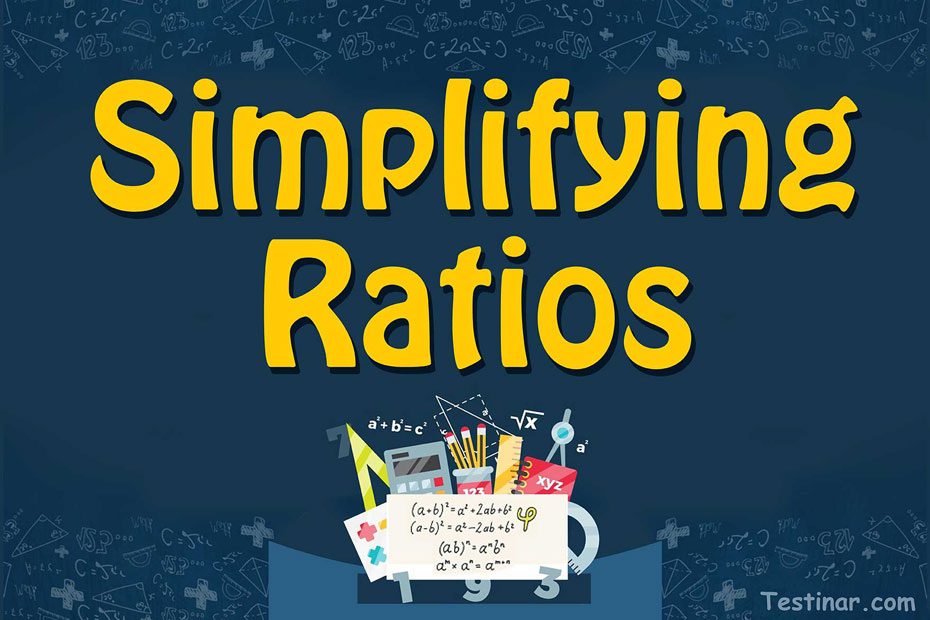## How to simplify ratios

What are Ratios- Definition!

In mathematics, the term "ratio" refers to the comparison of two numbers. This is typically done to determine how large or little a number or a quantity is in relation to another number or quantity. So, what approach do we employ in order to determine these ratios? In this case, we employ the division approach. When two numbers are divided, we have a ratio. The dividend component is referred to as the 'antecedent,' whereas the divisor component is referred to as the 'consequent.'
So, let's look at an illustration. Consider the following scenario: there are $$25$$ vehicles in a market, $$17$$ of which are cars and the remaining $$8$$ are bikes. As a result, the ratio of the cars to the bikes in that market would be $$17:8$$. Furthermore, in mathematical terms, this can be read as "$$17$$ is to $$8$$" or something like that.

How to Calculate Ratios

To understand the exact process of calculating ratios, let’s take up the following two problems.

Question1: Suppose, in an electronic shop, there are $$13$$ iPhones and $$17$$ iMacs. So, what is the ratio of iPhones and iMacs in that particular electronic shop?

• Firstly, identify the unique entities. In this case, $$13$$ iPhones and $$17$$ iMacs are unique entities.
• Next, write these in a fraction form. So, we write it as $$\frac{13}{17}$$
• Now, we need to check if this fraction can be further simplified or not. Here, it can’t be simplified further.
• So, the ratio of iPhones and iMacs in that particular electronic shop is $$13:17$$.

Question2: A library contains a total of $$100$$ books. Out of them, $$20$$ are of mathematics and the rest are of biology. What is the ratio of mathematics and biology books in that library?

• Firstly, identify the unique entities. In this case, $$20$$ mathematics books, and $$100 \ \ – \ \ 20 \ \ = \ \ 80$$ biology books are unique entities.
• Next, write these in a fraction form. So, we write it as $$\frac{20}{80}$$
• Now, we need to check if this fraction can be further simplified or not. Here, it can be simplified further to $$1:4$$.
• So, the ratio of maths books to that of biology books in the library is $$1:4$$.

Simplifying Ratios

Check the entities in the given problem for writing ratios. Then divide both sides of the ratio to simplify it further. Suppose there are $$14$$ apples and $$7$$ oranges. So, the ratio of apples to oranges would be $$\frac{14}{7} \ \ = \ \ 14:7 \ \ = \ \ 2:1$$.

### Exercises for Simplifying Ratios

1) $$7 : 42 =$$

2) $$44 : 88 =$$

3) $$6 : 30 =$$

4) $$64 : 80 =$$

5) $$8 : 52 =$$

6) $$15 : 21 =$$

7) $$14 : 35 =$$

8) $$54 : 69 =$$

9) $$6 : 45 =$$

10) $$24 : 68 =$$

1) $$7 : 42 = \color{red}{\frac{7 \ \div \ 7 }{ 42 \ \div \ 7}}$$$$\color{red}{= \frac{1 }{ 6}}$$
2) $$44 : 88 = \color{red}{44 \ \div \ 44 : 88 \ \div \ 44 }$$$$\color{red}{ = 1 : 2}$$
3) $$6 : 30 = \color{red}{6 \ \div \ 6 : 30 \ \div \ 6 }$$$$\color{red}{ = 1 : 5}$$
4) $$64 : 80 = \color{red}{64 \ \div \ 16 : 80 \ \div \ 16 }$$$$\color{red}{ = 4 : 5}$$
5) $$8 : 52 = \color{red}{8 \ \div \ 4 : 52 \ \div \ 4 }$$$$\color{red}{ = 2 : 13}$$
6) $$15 : 21 = \color{red}{15 \ \div \ 3 : 21 \ \div \ 3 }$$$$\color{red}{ = 5 : 7}$$
7) $$14 : 35 = \color{red}{14 \ \div \ 7 : 35 \ \div \ 7 }$$$$\color{red}{ = 2 : 5}$$
8) $$54 : 69 = \color{red}{\frac{54 \ \div \ 3 }{ 69 \ \div \ 3}}$$$$\color{red}{= \frac{18 }{ 23}}$$
9) $$6 : 45 = \color{red}{\frac{6 \ \div \ 3 }{ 45 \ \div \ 3}}$$$$\color{red}{= \frac{2 }{ 15}}$$
10) $$24 : 68 = \color{red}{24 \ \div \ 4 : 68 \ \div \ 4 }$$$$\color{red}{ = 6 : 17}$$

## Simplifying Ratios Quiz

### HSPT Math Practice Workbook

$25.99$14.99

### SAT Math Test Prep Bundle

$69.99$35.99

### CHSPE Math for Beginners 2022

$24.99$14.99

### FTCE Math in 10 Days

$24.99$12.99